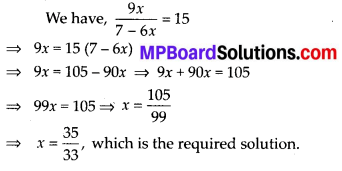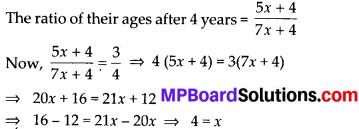# MP Board Class 8th Maths Solutions Chapter 2 Linear Equations in One Variable Ex 2.6

## MP Board Class 8th Maths Solutions Chapter 2 Linear Equations in One Variable Ex 2.6

Solve the following equations.

Question 1.
$$\frac{8 x-3}{3 x}=2$$
Solution:
We have, $$\frac{8 x-3}{3 x}$$ = 2
⇒ 8x – 3 = 2 × 3
⇒ 8x – 3 = 6x ⇒ 8x – 6x = 3 ⇒ 2x = 3
⇒ x = $$\frac{3}{2}$$, which is the required solution.

Question 2.
$$\frac{9 x}{7-6 x}=15$$
Solution:Question 3.
$$\frac{z}{z+15}=\frac{4}{9}$$
Solution:Question 4.
$$\frac{3 y+4}{2-6 y}=\frac{-2}{5}$$
Solution:Question 5.
$$\frac{7 y+4}{y+2}=\frac{-4}{3}$$
Solution:Question 6.
The ages of Hari and Harry are in the ratio 5 : 7. Four years from now the ratio of their ages will be 3 : 4. Find their present ages.
Solution:
Let the present ages of Hari and Harry be 5x and 7x respectively.
After 4 years, Hari’s age = (5x + 4) years and Harry’s age = (7x + 4) years∴ Hari’s present age = 5x = 5 × 4 = 20 years and Harry’s present age = 7x = 7 × 4 = 28 yearsQuestion 7.
The denominator of a rational number is greater than its numerator by 8. If the numerator is increased by 17 and the denominator is decreased by 1, the number
obtained is $$\frac{3}{2}$$. Find the rational number.
Solution:
Let the numerator of the rational number be x.
∴ The denominator is x + 8.
According to question, we have
New numerator = x + 17 and
New denominator = (x + 8) – 1 = x + 7⇒ 2(x + 17) = 3(x + 7) ⇒ 2x + 34 = 3x + 21
⇒ 34 – 21 = 3x – 2x ⇒ 13 = x
Thus, numerator = x = 13
and denominator = x + 8 = 13 + 8 = 21
Hence, the rational number is $$\frac{13}{21}$$.